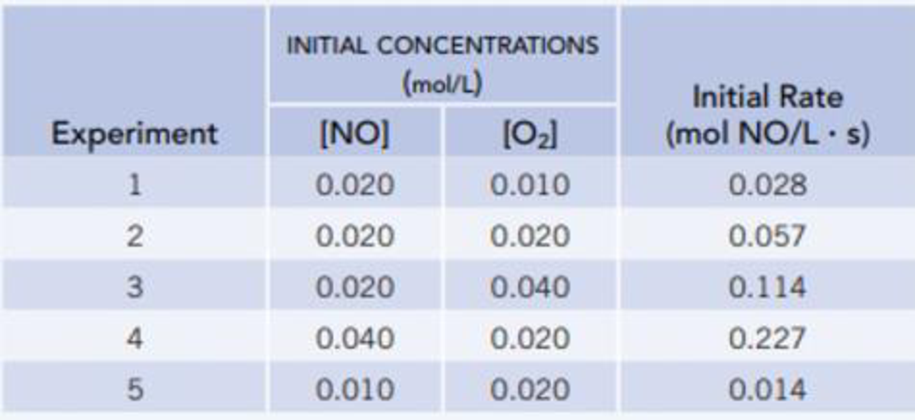Chapter 14.3, Problem 14.3CYU

Chapter
Section
Textbook Problem

The initial rate (‒ Δ[NO]/ Δt] of the reaction of nitrogen monoxide and oxygenNO(g) + ½2O2(g) → NO2(g)was measured for various initial concentrations of NO and O2 at 25 °C. Determine the rate equation from these data. What is the value of the rate constant, k, and what are its units?Interpretation Introduction

Interpretation: The reaction rate and the value of rate constant has to be given.

Concept introduction:

Rate law or rate equation: The relationship between the reactant concentrations and reaction rate is expressed by an equation.

aA + bBxXRate of reaction = k [A]m[B]n

Order of a reaction: The order of a reaction with respect to a particular reactant is the exponent of its concentration term in the rate law expression, and the overall reaction order is the sum of the exponents on all concentration terms.

Rate constant, k: It is a proportionality constant that relates rate and concentration at a given temperature.

Explanation

The reaction rate of the chemical reaction is given as,

Reaction Rate = k [NO]m[O2]n,where m, and n are orders of the reactants.Givenreaction: NO(g)+ 1/2 O2(g)®NO2(g)Orderofthereaction:_*Comparingfirsttwoexperiments1and2,rate1=[NO]1m[O2]1n, rate1 = 0.028 mol/L.hrate2 = k [NO]2m[O2]2n, rate 2 = 0.057 mol/L.hrate2rate1=k [NO]2m[O2]2nk [NO]1m[O2]1n0.057mol/L.h0.028mol/L.h=(0.020)m(0.020)n(0.020)m(0.010)n 2.0 = (2)nn = 1Comparinglasttwoexperiments2and4,rate2 =[NO]2m[O2]2n,rate 2 = 0.057 mol/L.hrate4 = k [NO]4m[O2]4n,rate 4 = 0.227 mol/L.hrate 4rate2 =k [NO]4m[O2]4nk [NO]2m[O2]2n0

Still sussing out bartleby?

Check out a sample textbook solution.

See a sample solution

The Solution to Your Study Problems

Bartleby provides explanations to thousands of textbook problems written by our experts, many with advanced degrees!

Get Started